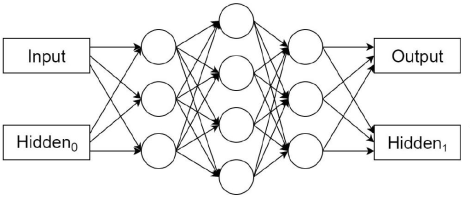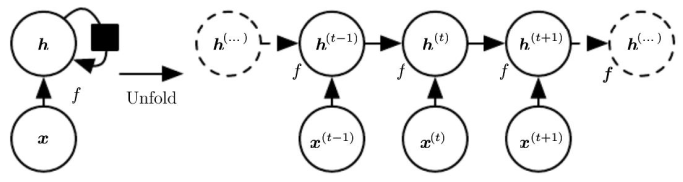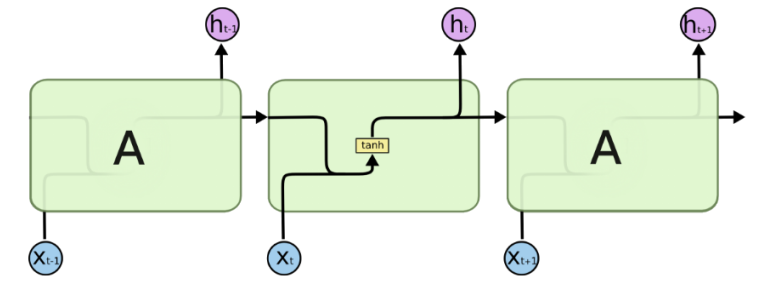# Pytorch实现基本循环神经网络RNN

## RNN

Recurrent Neural networks(Rumelhart, 1986) 主要用来处理序列型数据，具有对以往数据的记忆功能。下图所示，输入为input和一个状态Hidden0，输出为output和Hidden1.h t = tanh ⁡ ( w i h x t + b i h + w h h h t − 1 + b h h ) h_{t}=\tanh(w_{ih}x_{t}+b_{ih}+w_{hh}h_{t-1}+b_{hh})

• input_size：输入 x x 的特征数量
• hidden_size：隐藏状态 h h 的特征数量
• num_layers:网络层数，默认为1层。若2层，两个RNN单元进行堆叠，第2个RNN单元将第1个RNN单元的 h h 作为输入。
• nonlinearity：实用tanh或ReLU，默认是tanh
• bias：False代表不使用偏置 b i h b_{ih} b h h b_{hh} ，默认为True。
• batch_first:决定输入数据的维度顺序。默认输入是(seq,batch,feature)，分别是RNN序列长度，批量数，特征维度。True表示(batch,seq,feature).
• dropout:接收一个0~1的数值，会在网络除最后一层之外的其他输出层加上dropout层，默认为0
• bidirectional：如果是True，表示双向的RNN。

RNN接受一个序列输入 x t x_{t} with维度(seq,batch,feature)和记忆输入 h 0 h_{0} with维度(num_layers*num_directions,batch,hidden)，num_layers表示RNN单元堆叠的层数，num_directions为1(单向RNN)和2（双向RNN），双向RNN就是从左向右计算一次之后再从右向左计算一次，hidden就是输出维度。网络输出为output和 h n h_{n} 。output表示每个时刻网络最后一层的输出，维度是(seq,batch,hidden*num_directions)，即将双向的特征拼接起来。 h n h_{n} 是最后时刻所有堆叠的记忆单元的所有输出，维度为(num_layers*num_directions, batch,hidden)

>>> import torch.nn as nn
>>> rnn = nn.RNN(10, 20, 2)
>>> input = torch.randn(5, 3, 10)
>>> h0 = torch.randn(2, 3, 20) # h0不初始化则全部为0
>>> output, hn = rnn(input, h0)


• RNN.weight_ih_l[k]： 表示第 k k 层的input-hidden权重。如果 k = 0 k=0 ，表示第0层维度为(hidden_size,input_size),其他层的维度为(hidden_size,num_directions*hidden_size)，接收第 k − 1 k-1 层传来的hidden特征。
• RNN.weight_hh_l[k]: 表示第 k k 层的hidden-hidden权重，维度是(hidden_size,hidden_size)，接收第 t − 1 t-1 时刻第 k k 层的hidden特征。
• RNN.bias_ih_l[k]: 表示第 k k 层的input-hidden的偏置，维度为(hidden_size)
• RNN.bias_hh_l[k]: 表示第 k k 层的hidden-hidden的偏置，维度为(hidden_size)
>>> rnn.weight_ih_l0.size()
torch.Size([20, 10])
>>> rnn.weight_ih_l1.size()
torch.Size([20, 20])
>>> rnn.weight_hh_l0.size()
torch.Size([20, 20])
>>> rnn.weight_hh_l1.size()
torch.Size([20, 20])


RNN的缺点：

11-23148112-06524
08-061万+
08-081873
02-129491
07-07438
03-039347
03-211万+
03-2227
04-223321
07-061720
02-2059
09-022317
12-224479
10-31276
07-05302
01-022787
06-161106
©️2020 CSDN 皮肤主题: 编程工作室 设计师:CSDN官方博客